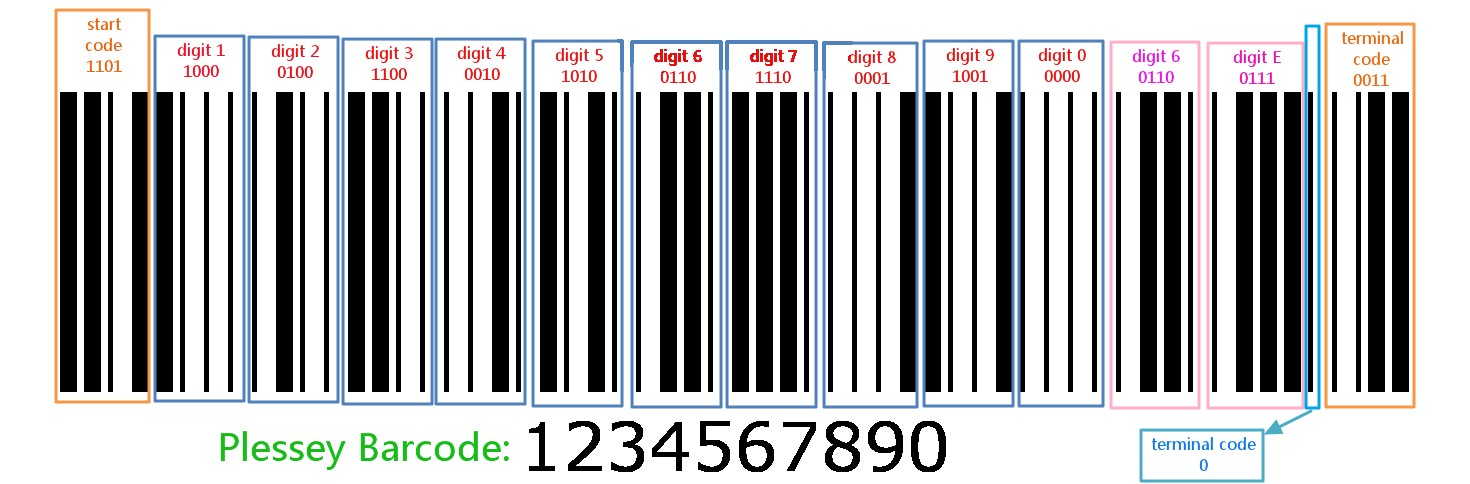## 1.2. Plessey Barcode Format

### 1.2.1. An Example

Figure 1.1. Plessey Barcode Format ExampleFigure 1.2. Plessey Barcode “01234567890” Example### 1.2.2. How to represent bits/digits/characters

Plessey’s barcode pattern is so simple that, with casual familiarity with the code, you can actually read the bar codes with your eyes.

Plessey basic code rule is:

• Wide bar following by narrow space is a "1" bit.
• Narrow bar followed by wide space is a "0" bit.

And each digit number is represented by four bit.

If you only calculate the bar (horizontal black line), the wide bar means ‘1’ and the narrow bar means ‘0’.

So, the digit ‘1’:

• BCD (Binary- Coded Decimal) is 0001b
• reversed BCD is 1000b
• wide bar + narrow bar + narrow bar + narrow bar

### 1.2.3. Barcode Format

Table 1.1. Plessey Barcode Format

start character Any number of labels/characters/digits Checksum / check digit / code A stop / termination character / block / pitch bar the reverse start code
4it =1101 XXX...(eg, 012345...) 8bit(eg. 0110 0111) 1bit=X(eg, 1) 4bit=0011

In which:

• the forward start code

The forward start code is "1101". It defines the beginning of the encoded text.

• the label / data digits

The characters of the text to encode are hexadecimal values encoded as reversed BCD.

Table 1.2. Plessey Bits Encoding Rule

Value Encoding Value Encoding Value Encoding Value Encoding
0 0000 4 0010 8 0001 C 0011
1 1000 5 1010 9 1001 D 1011
2 0100 6 0110 A 0101 E 0111
3 1100 7 1110 B 1101 F 1111

• the check code, for error detection

The check code for error detection and correction is as CRC, using polynomial division.

Is uses 2 characters, or 8 bits.

The generator polynomial is g(x) = x8 + x7 + x6 + x5 + x3 + 1 with n=8, in binary "111101001".

• the termination bar

The termination bar follows the CRC. It's a full pitch bar.

• the reverse start code

The reverse start code, with the forward start code, are used to detect the direction of reading : from left to right or right to left.

It's encoded as a reversed "0011".

Reversed because the "0" is represented by a wide space and simple bar, and "1" as a simple space and wide bar.